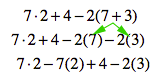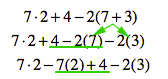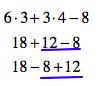Home > CC2 > Chapter 4 > Lesson 4.2.2 > Problem4-44

4-44.

Identify the mathematical property, operation, or idea that justifies each sequence of expressions below. Then find the value of the expression. Homework Help ✎

1. $7·2+4−2(7+3)$
$7·2+4−2(7)−2(3)$
$7·2−7(2)+4−2(3)$Notice how the Distributive Property was used to change the first line into the second.

Two terms were moved using the Commutative Property of Addition. Keep in mind that subtraction is the same as adding a negative. Also, note that the term $2(7)$ became $7(2)$ through the Commutative Property of Multiplication.

Simplify each term, then add them together. Circle the terms if it helps.
$7·2−7(2)+4−2(3)=$
$14−14+4−6=$

$-2$

1. $6·3+3·4−8$
$18+12−8$
$18−8+12$

Look at the differences between each line carefully. What was done to change the first line into the second? What property was used to move the underlined terms?$18−8+12=22$
Make sure you identify mathematical properties, operations, and ideas that are used.

1. $3^2+5(1−3)+4·5+1$
$3^2+4·5+1+5(−2)$
$9+20+1−10$

Be very careful with this one. Multiple properties and operations are used in each line.

1. $(8+(−12))+10+2$
$8+(−12+10+2)$

Only one property is used in this one.